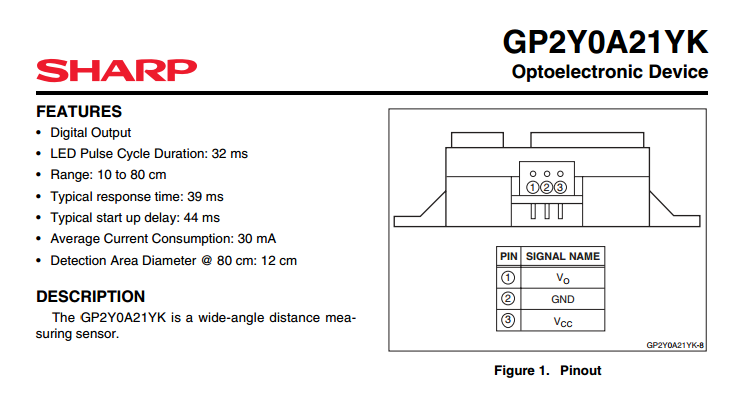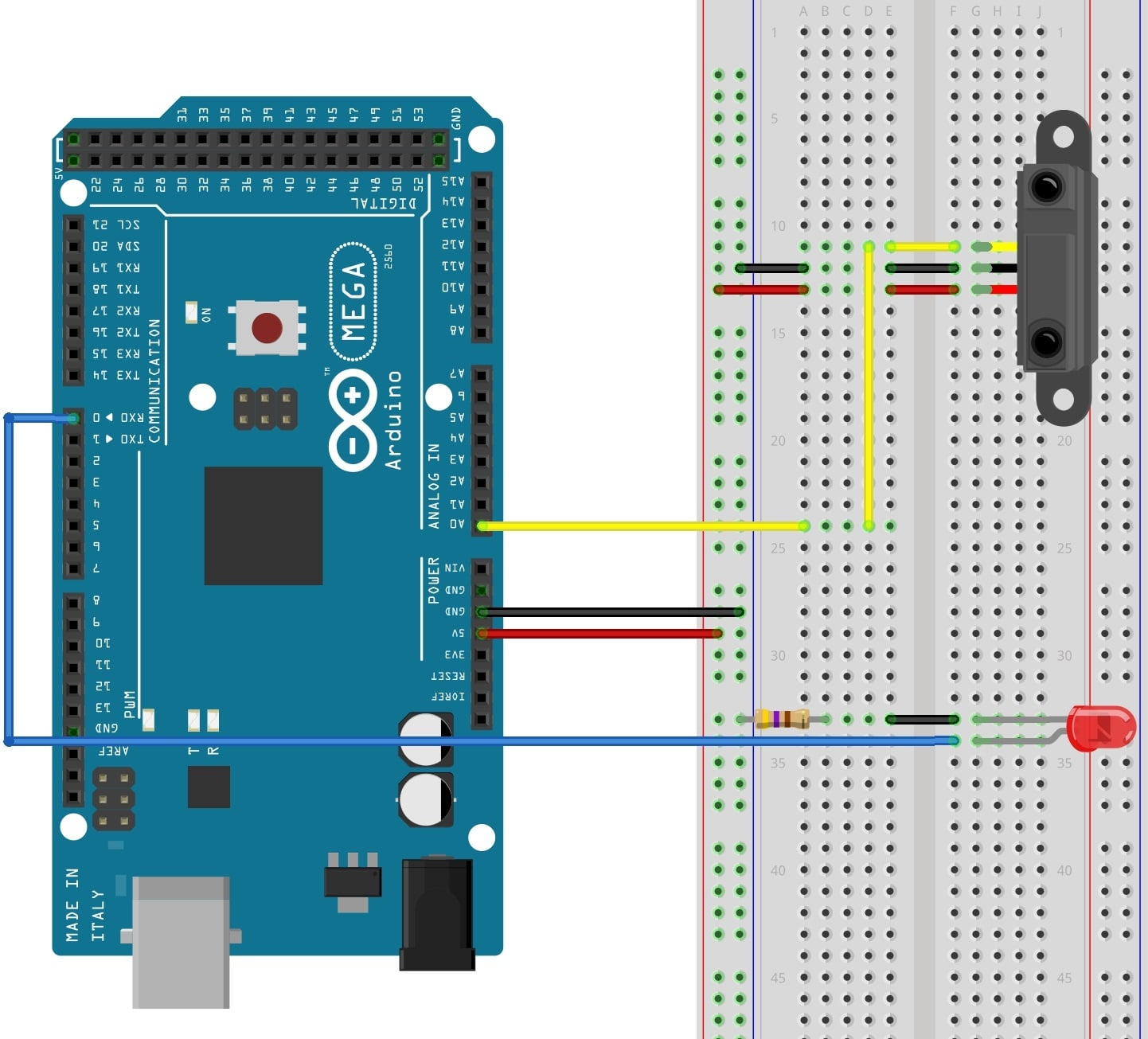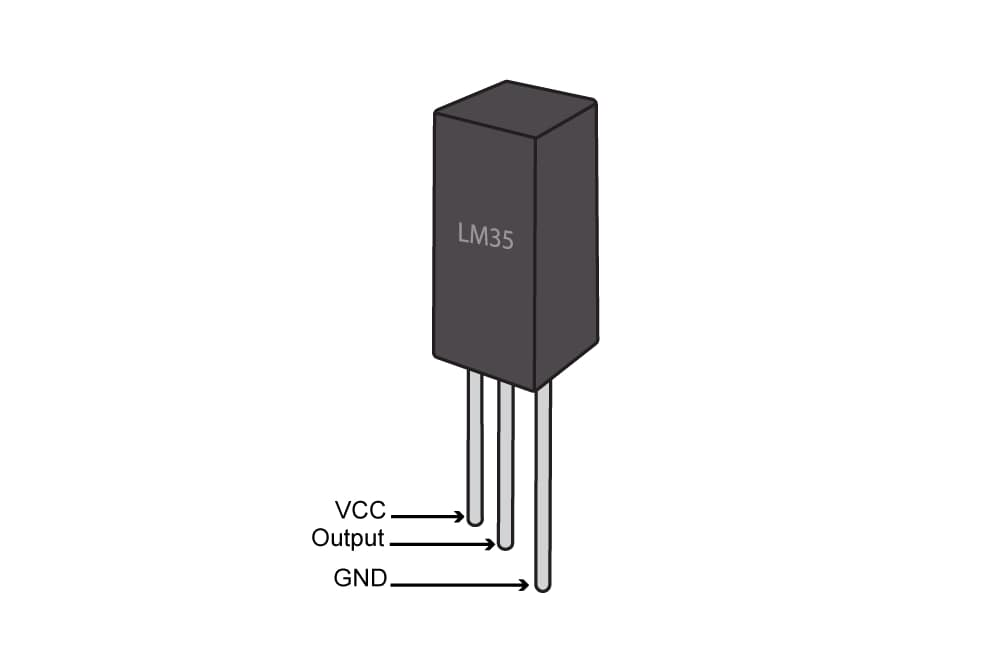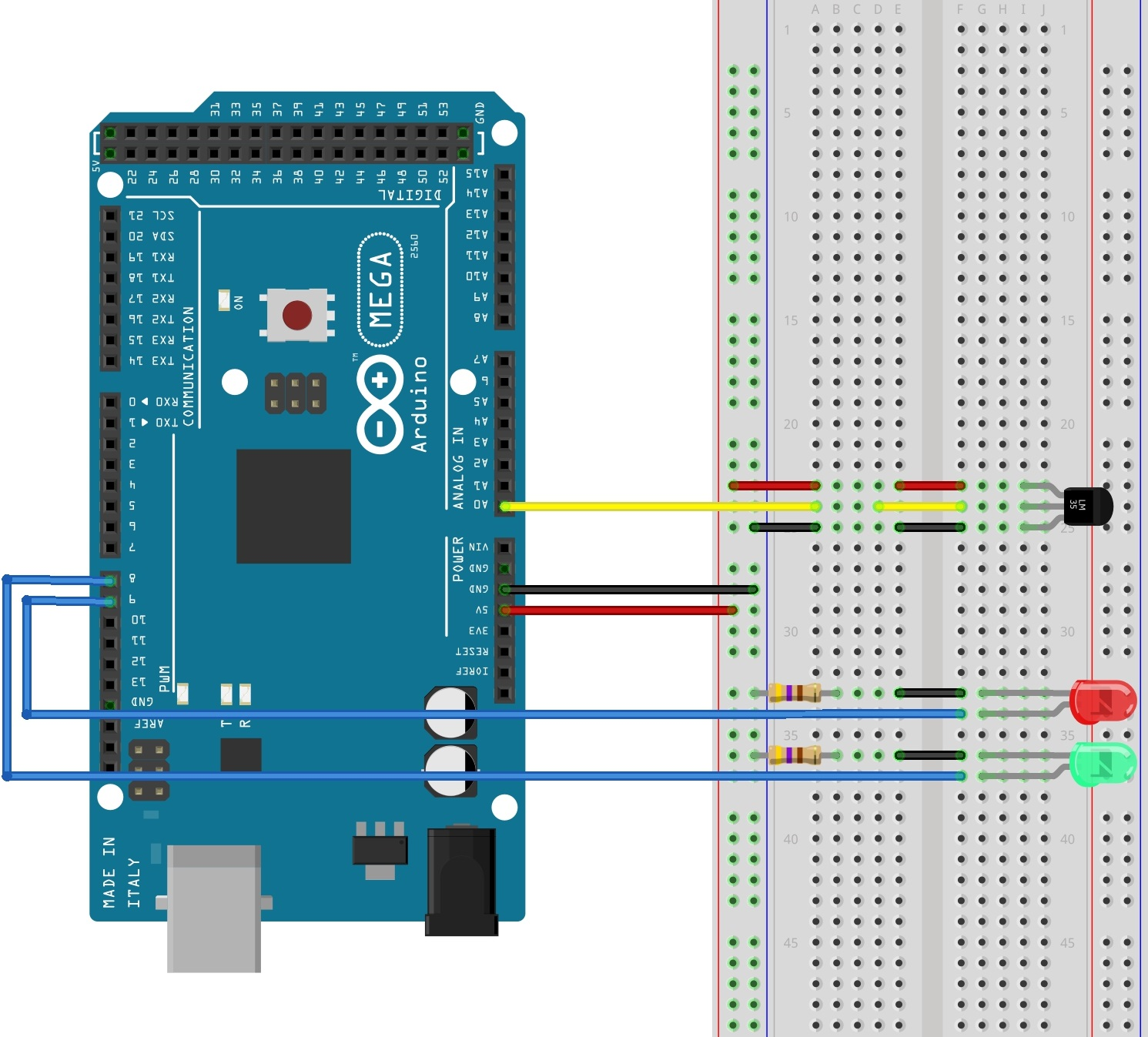Project

# Reading Sensors with an Arduino

June 18, 2015 by Tim Youngblood

## The ease with which an Arduino can obtain sensor values is one of the features that makes it so useful.

The ease with which an Arduino can obtain sensor values is one of the features that makes it so useful.

Sensors are devices that convert a physical quantity, such as light intensity or temperature, into an electrical quantity. A thermocouple, for example, outputs a voltage proportional to its temperature. There are many different types of sensors:

• Light  sensor
• Motion  sensor
• Temperature  sensor
• Magnetic fields  sensor
• Gravity  sensor
• Humidity  sensor
• Moisture  sensor
• Vibration  sensor
• Pressure  sensor
• Electrical fields sensor
• Sound sensor
• Position sensor

These sensors are used in thousands of different applications, including manufacturing, machinery, aerospace, automobiles, medicine, and robotics.

#### EXPERIMENT 1: Distance sensor

In this experiment, we will use a Sharp GP2Y0A21YK proximity sensor to control the brightness of an LED.SHARP IR Sensor

#### Hardware Required

• 1 x Arduino Mega2560
• 1 x LED
• 5 x jumper wires
• 1 x 470 ohm resistor
• 1 X Sharp GP2Y0A21YK proximity sensor

#### Wiring DiagramCircuit Diagram

The Sharp proximity sensor can detect objects as close as 10 cm and as far away as 80 cm. It emits a pulse of infrared light and then detects the angle at which that light is reflected. The farther away an object is, the lower the output voltage. If the sensor receives no reflection, the output voltage of the sensor will be 0 V. If the object is 10 cm or closer, the output voltage will be equal to 5 V. (In this experiment, we are supplying 5V to the sensor.)

The sensor's output is connected to an Arduino analog input. The Arduino's analog-to-digital converter (ADC) then converts that value to a value between 0 and 1023. This value is then mapped to a value between 0 and 255, and that number is used to set the duty cycle of a pulse-width modulated output, which controls the brightness of the LED. The result is that the closer an object is to the proximity sensor, the brighter the LED will shine.

#### Code For Experiment 1

const int pwm = 2  ;  //Initializing Pin for pwm

void setup()
{
pinMode(pwm,OUTPUT) ;  // To change LED brightness
}
void loop()
{
sensor_val = map(sensor_val, 0, 1023, 0, 255) ;

/*
-----------map funtion------------
The above funtion scales the output of adc,which is
10 bit and gives values btw 0 to 1023, in values btw
0 to 255 form analogWrite funtion which only recieves
values btw this range.
*/

analogWrite(pwm,sensor_val) ; // setting sensor value as pwm

}

EXPERIMENT 2: Heat Sensor

In this experiment, the Arduino will measure the temperature using an LM35 sensor IC. The LM35 is a low voltage IC which requires a power supply from +4 VDC to +20 VDC. This is ideal because we can power the sensor with the Arduino's +5 V output. The LM35 has just 3 pins, 2 for the power supply and one for the analog output. The output pin provides an analog voltage output that is linearly proportional to the temperature in degrees C. The output ranges from 0 V - 1.5 V, when powered with a single power supply. An output of 0 V corresponds to a temperature of 0 degrees C, and the output increases 10 mV for every degree increase in the temperature. To convert the output voltage to temperature, you need only to divide the output voltage in mV by 10. For example, if the output value equals 315 mV (0.315 V), the temperature is 31.5°C.

Pin Configuration of LM35 IC:Pin Configuration

​

Hardware Required

1 x LM35 Temperature Sensor
2 x LEDs
1 x matchbox
2 X 470 ohm resistors
1 x Arduino Mega2560
10 x jumper wires

Wiring DiagramCircuit Diagram

​

Code For Experiment 2

The LM35's output pin (pin 2) is connected to A0 of the Arduino. The code uses the function analogRead() to convert the output voltage to a number between 0 and 1023. Multiplying this number by 0.48828125 converts that value to degrees C, which is then displayed on the serial monitor:

const int adc = 0 ; //naming pin 0 of analog input side as adc
const int high = 8 ; // For turning on and off yellow LED
const int low = 9 ; // For turning on and off Green LED
void setup()
{
Serial.begin(9600) ; //Starting serial Communication at baud rate of 9600
pinMode(high,OUTPUT); //declaring LED pins as OUTPUT
pinMode(low,OUTPUT);
}
void loop()
{
Serial.print("TEMPRATURE = "); //to Display on serial monitor
Serial.print("*C"); //TEMPRATURE = 27*C ETC
Serial.println(); //To end the line
delay(1000); //1 Sec delay
/*
LOGIC:
if (temperature (adc) > 70 ° C )
turn on Yellow Leds
turn off Green Leds
else
turn off Yellow Leds
turn on Green Led
*/
if(adc>70) // This is the control statement
{
digitalWrite(high,HIGH) ;
digitalWrite(low,LOW) ;
}
else
{
digitalWrite(high,LOW) ;
digitalWrite(low,HIGH) ;
}